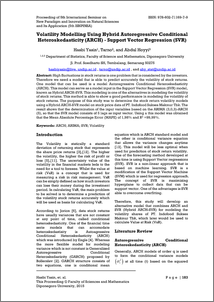# Volatility Modelling Using Hybrid Autoregressive Conditional Heteroskedasticity (ARCH) - Support Vector Regression (SVR)

Yasin, Hasbi and Tarno, T. and Hoyyi, Abdul (2015) Volatility Modelling Using Hybrid Autoregressive Conditional Heteroskedasticity (ARCH) - Support Vector Regression (SVR). In: The 5th International Seminar on New Paradigm and Innovation on Natural Sciences and Its Application (5th ISNPINSA) i , 7-8 Oktober 2015, Semarang.Preview
PDF - Published Version
663Kb

## Abstract

High fluctuations in stock returns is one problem that is considered by the investors. Therefore we need a model that is able to predict accurately the volatility of stock returns. One model that can be used is a model Autoregressive Conditional Heteroskedasticity (ARCH). This model can serve as a model input in the Support Vector Regression (SVR) model, known as Hybrid ARCH-SVR. This modeling is one of the alternatives in modeling the volatility of stock returns. This method is able to show a good performance in modeling the volatility of stock returns. The purpose of this study was to determine the stock return volatility models using a Hybrid ARCH-SVR model on stock price data of PT. Indofood Sukses Makmur Tbk. The result shows that the determination of the input variables based on the ARIMA (3,0,3)-ARCH (5), so that the SVR model consists of 5 lags as input vector. Using a this model was obtained that the Mean Absolute Percentage Error (MAPE) of 1,98% and R2 =99,99%.

Item Type: Conference or Workshop Item (Paper) Q Science > Q Science (General) Faculty of Science and Mathematics > Department of Chemistry 62543 INVALID USER 28 May 2018 09:23 28 May 2018 09:23

Repository Staff Only: item control page